Advertisement Remove all ads

# Write the Steps in Deducing the Chemical Formulae of the Following Compounds. Sodium Sulphate, Potassium Nitrate, Ferric Phosphate, Calcium Oxide, Aluminium Hydroxide - Science and Technology

Answer in Brief

Write the steps in deducing the chemical formulae of the following compounds.

Sodium sulphate, potassium nitrate, ferric phosphate, calcium oxide, aluminium hydroxide

Advertisement Remove all ads

#### Solution

a.Sodium sulphate :
Step 1 : Write the symbols of the radicals.
Na                         SO4
Step 2 : Write the valency below the respective radical.
Na                         SO4
1                             2
Step 3 : Cross-multiply symbols of radicals with their respective valency.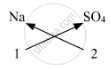Step 4 : Write down the chemical formula of the compound.

Na2SO4

b. Potassium nitrate :
Step 1 : Write the symbols of the radicals
K                              NO3

Step 2 : Write the valency below the respective radical.
K                              NO3
1                                1
Step 3 : Cross-multiply symbols of radicals with their respective valency.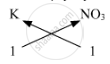Step 4 : Write down the chemical formula of the compound.

KNO3

c. Ferric phosphate :
Step 1 : Write the symbols of the radicals
Fe                             PO4

Step 2 : Write the valency below the respective radical.
Fe                             PO4
3                               3
Step 3 : Cross-multiply symbols of radicals with their respective valency.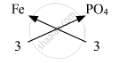Step 4 : Write down the chemical formula of the compound.

FePO4

d. Calcium oxide :
Step 1 : Write the symbols of the radicals
Ca                             O

Step 2 : Write the valency below the respective radical.
Ca                             O
2                               2

Step 3 : Cross-multiply symbols of radicals with their respective valency.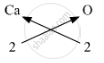Step 4 : Write down the chemical formula of the compound.

CaO

e. Aluminium hydroxide :
Step 1 : Write the symbols of the radicals
Al                             OH

Step 2 : Write the valency below the respective radical.
Al                             OH
3                               1

Step 3 : Cross-multiply symbols of radicals with their respective valency.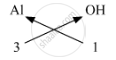Step 4 : Write down the chemical formula of the compound.

Al(OH)3

Concept: Chemical Formulae of Compound : Recapitulation
Is there an error in this question or solution?
Advertisement Remove all ads

#### APPEARS IN

Balbharati Science and Technology 9th Standard Maharashtra State Board
Chapter 4 Measurement of Matter
Exercise | Q 3 | Page 57
Advertisement Remove all ads
Advertisement Remove all ads
Share
Notifications

View all notifications

Forgot password?